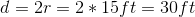# ISEE Upper Level Math : How to find the diameter of a sphere

## Example Questions

### Example Question #1 : Spheres

There is a perfectly spherical weather balloon with a surface area of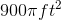, what is its diameter?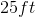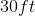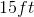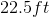Explanation:

There is a perfectly spherical weather balloon with a surface area of, what is its diameter?

Begin with the formula for surface area of a sphere: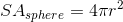Now, set it equal to the given surface area and solve for r: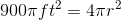First divide both sides by.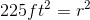Then square root both sides to get our radius: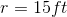Now, because the question is asking for our diameter and not our radius, we need to double our radius to get our answer: